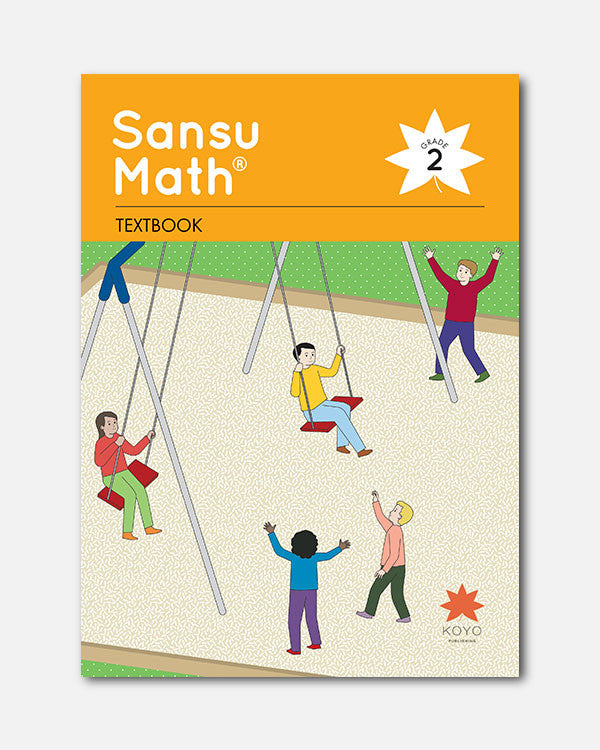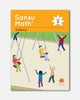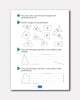# Sansu Math® Textbook 2

In Stock
\$ 25.00

### Overview

Sansu Math® Grade 2 is the second book in the five-book Sansu Math® elementary mathematics series. The material includes 20 units in one textbook, which includes the same student and shape characters used throughout the series.

1. Tables and Graphs
2. Time and Elapsed Time
3. Properties of Addition
4. Subtraction Algorithm
1. Subtraction (1)
2. Subtraction (2)
3. Properties of Subtraction
5. Units of Length
1. How to Express and Measure Length
2. Units of Length
3. Calculation of Length
6. 3-Digit Numbers
1. How to Express Numbers and Their Structure
2. Calculation with Tens and Hundreds
3. Comparing Numbers
7. Units of Money
8. Better Ways to Calculate
1. Calculation of Three Numbers
2. Addition and Subtraction
9. Addition and Subtraction Algorithms
2. Subtraction Algorithm
3. Algorithms for Calculations with Large Numbers
10. Triangles and Quadrilaterals
1. Triangles and Quadrilaterals
2. Rectangles and Squares
11. Fractions
12. Multiplication (1)
1. Multiplication
2. Multiplication Facts: 5 and 2
3. Multiplication Facts: 3 and 4
13. Multiplication (2)
1. Multiplication Facts: 6 and 7
2. Multiplication Facts: 8, 9 and 1
3. Times as Much and Multiplication
4. The Multiplication Table and Its Properties
5. Problems
14. 4-Digit Numbers
15. Units of Length of Long Objects
16. U.S. Customary Units of Length
17. Units of Capacity
18. U.S. Customary Units of Capacity
19. Addition and Subtraction
20. Shapes of Boxes